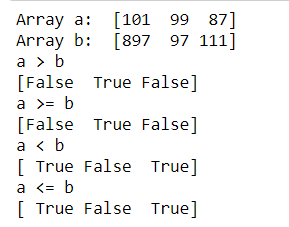# How to compare two NumPy arrays?

## How to compare two NumPy arrays?

This article focuses on the comparison done using NumPy on arrays. Comparing two NumPy arrays determines whether they are equivalent by checking if every element at each corresponding index are the same.

Method 1:

We generally use the `==` operator to compare two NumPy arrays to generate a new array object. Call ndarray.all() with the new array object as ndarray to return True if the two NumPy arrays are equivalent.

 `import` `numpy as np` ` ` `an_array ``=` `np.array([[``1``, ``2``], [``3``, ``4``]])` `another_array ``=` `np.array([[``1``, ``2``], [``3``, ``4``]])` ` ` `comparison ``=` `an_array ``=``=` `another_array` `equal_arrays ``=` `comparison.``all``()` ` ` `print``(equal_arrays)`

Output:

`True`

Method 2:

We can also use greater than, less than and equal to operators to compare. To understand, have a look at the code below.

```Syntax : numpy.greater(x1, x2[, out])
Syntax : numpy.greater_equal(x1, x2[, out])
Syntax : numpy.less(x1, x2[, out])
Syntax : numpy.less_equal(x1, x2[, out])```
 `import` `numpy as np` ` ` ` ` `a ``=` `np.array([``101``, ``99``, ``87``])` `b ``=` `np.array([``897``, ``97``, ``111``])` ` ` `print``(``"Array a: "``, a)` `print``(``"Array b: "``, b)` ` ` `print``(``"a > b"``)` `print``(np.greater(a, b))` ` ` `print``(``"a >= b"``)` `print``(np.greater_equal(a, b))` ` ` `print``(``"a < b"``)` `print``(np.less(a, b))` ` ` `print``(``"a <= b"``)` `print``(np.less_equal(a, b))`

Output:Last Updated on March 17, 2022 by admin

## How to calculate dot product of two vectors in Python?How to calculate dot product of two vectors in Python?

How to calculate dot product of two vectors in Python? In mathematics, the dot product or also

## Three-dimensional Plotting in Python using MatplotlibThree-dimensional Plotting in Python using Matplotlib

Three-dimensional Plotting in Python using Matplotlib Matplotlib was introduced keeping in mind, only two-dimensional plotting. But

## Django FormsDjango Forms

Django Forms When one creates a Form class, the most important part is defining the fields of

Reading CSV files in Python A CSV (Comma Separated Values) file is a form of plain text document

## How to change the Tkinter label text?How to change the Tkinter label text?

How to change the Tkinter label text?   Tkinter is a standard GUI (Graphical user interface)

## Generating all possible Subsequences using RecursionGenerating all possible Subsequences using Recursion

Generating all possible Subsequences using Recursion Given an array. The task is to generate and

## How to open and close a file in PythonHow to open and close a file in Python

How to open and close a file in Python There might rise a situation where